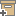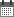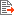ניהול
קהילה:
אסיף מאגר המחקר החקלאי
The spatial variability of water and solute transport properties in unsaturated soil: II. Scaling models of water transportBack to searchPrevious item
Next item
Year:
1987
Source of publication :
Hilgardia
Authors :
רוסו, דוד
;
.
Volume :
55
Co-Authors:

William A. Jury
David Russo
Garrison Sposito

Facilitators :
From page:
33
To page:
56
(
Total pages:
24
)
Abstract:

In this paper, we examine the possibility of introducing a single stochastic scaling factor α, derived from macroscopic Miller similitude, to describe the spatial variability of soil hydraulic properties. Most of the information available allowed only a conventional statistical analysis of the scaling factors derived from different soil properties. The field studies of (Nielsen, Biggar, and Erh (1973)) and (Russo and Bresler (1981)) were suitable also for more detailed structural analyses. Results of these analyses suggested that the spatial structure of the α-set derived from the hydraulic conductivity function K(?) is different from that of the α-set derived from the water retentivity function h(?), reflecting the different spatial structures of the K(?) and the h(?) functions. Consequently, the statistical relationship between the uncorrelated residuals of the two α-sets was rather weak. For the Hamra field of (Russo and Bresler (1981)), the use of relative hydraulic properties to estimate the scaling factor sets considerably improved the correlation between the α-sets, which had essentially the same spatial structure but slightly different variances.

In this study, where the soil hydraulic properties are assumed to be described by the model of (Brooks and Corey (1964)), analytical expressions for the variances of the two different α-sets indicated that (1) both α-sets are dependent on the range of water saturation that is used to estimate them, (2) the correlation between the two sets will improve in media with a wide pore-size distribution, and (3) the two sets will be identical if and only if the relative hydraulic conductivity function Kr(hr) is described by a deterministic function, Kr(hr) = hr-2. This result suggested that, in general, a second scaling factor for Kr is required for media that are not characterized by this single deterministic relationship.

A more general Kr(hr) relation, defined by Kr = hr-?;, was introduced using ?; as a second stochastic variable. In this representation, the α scaling factor for Kr is defined by Kr/Kr* = α?; instead of α2 as in macroscopic Miller similitude. For the Hamra field, the resultant new α-set was identical to the α-set derived from the relative retentivity function. For the Panoche field, using the values of ?; to estimate the scaling factor from the relative hydraulic conductivity function considerably improved the correlation and the similarity between the two α-sets, but did not render them identical. The results of our analysis suggest that, for transient water flow, describing the spatial variability of K(?) and h(?) requires at least three stochastic variates: Ks, α, and ?;

Note:
Related Files :
solute transport properties
spatial variability of water
Unsaturated soil
עוד תגיות
תוכן קשור
More details
DOI :
10.3733/hilg.v55n04p025
Article number:
0
Affiliations:
Database:
Publication Type:
מאמר
;
.
Language:
אנגלית
Editors' remarks:
ID:
55950
Last updated date:
02/03/2022 17:27
Creation date:
18/08/2021 20:23Scientific Publication
The spatial variability of water and solute transport properties in unsaturated soil: II. Scaling models of water transport
55

William A. Jury
David Russo
Garrison Sposito

The spatial variability of water and solute transport properties in unsaturated soil: II. Scaling models of water transport

In this paper, we examine the possibility of introducing a single stochastic scaling factor α, derived from macroscopic Miller similitude, to describe the spatial variability of soil hydraulic properties. Most of the information available allowed only a conventional statistical analysis of the scaling factors derived from different soil properties. The field studies of (Nielsen, Biggar, and Erh (1973)) and (Russo and Bresler (1981)) were suitable also for more detailed structural analyses. Results of these analyses suggested that the spatial structure of the α-set derived from the hydraulic conductivity function K(?) is different from that of the α-set derived from the water retentivity function h(?), reflecting the different spatial structures of the K(?) and the h(?) functions. Consequently, the statistical relationship between the uncorrelated residuals of the two α-sets was rather weak. For the Hamra field of (Russo and Bresler (1981)), the use of relative hydraulic properties to estimate the scaling factor sets considerably improved the correlation between the α-sets, which had essentially the same spatial structure but slightly different variances.

In this study, where the soil hydraulic properties are assumed to be described by the model of (Brooks and Corey (1964)), analytical expressions for the variances of the two different α-sets indicated that (1) both α-sets are dependent on the range of water saturation that is used to estimate them, (2) the correlation between the two sets will improve in media with a wide pore-size distribution, and (3) the two sets will be identical if and only if the relative hydraulic conductivity function Kr(hr) is described by a deterministic function, Kr(hr) = hr-2. This result suggested that, in general, a second scaling factor for Kr is required for media that are not characterized by this single deterministic relationship.

A more general Kr(hr) relation, defined by Kr = hr-?;, was introduced using ?; as a second stochastic variable. In this representation, the α scaling factor for Kr is defined by Kr/Kr* = α?; instead of α2 as in macroscopic Miller similitude. For the Hamra field, the resultant new α-set was identical to the α-set derived from the relative retentivity function. For the Panoche field, using the values of ?; to estimate the scaling factor from the relative hydraulic conductivity function considerably improved the correlation and the similarity between the two α-sets, but did not render them identical. The results of our analysis suggest that, for transient water flow, describing the spatial variability of K(?) and h(?) requires at least three stochastic variates: Ks, α, and ?;

Scientific Publication
You may also be interested in